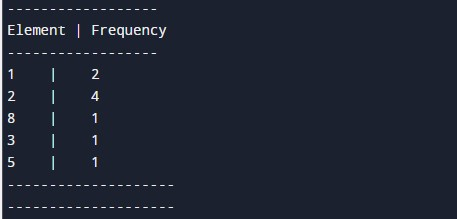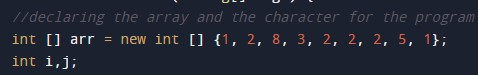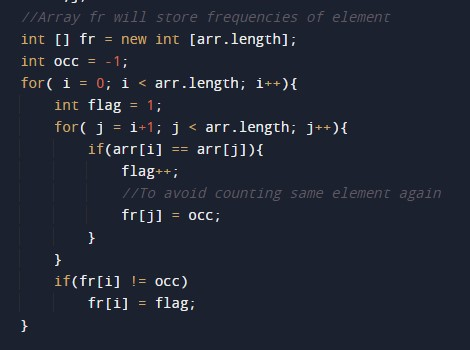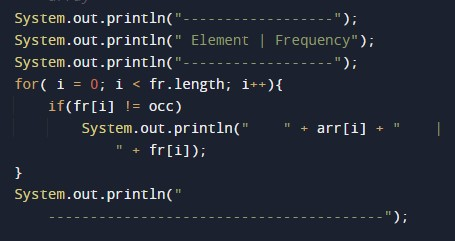# Java Program to find the frequency of each element in the array

In this tutorial you will learn about the Java Program to find the frequency of each element in the array and its application with practical example.

## Java Program to find the frequency of each element in the array

In this tutorial, we will learn to create a Java program that will find the Frequency of each Element in an Array using Java programming.

## Prerequisites

Before starting with this tutorial, we assume that you are the best aware of the following Java programming topics:

• Operators in Java Programming.
• Basic Input and Output function in Java Programming.
• Basic Java programming.
• For loop in Java programming.
• Arithmetic operations in Java Programming.

## Program to Find Frequency of each Element in an Array.

In this program, we will count the frequency of each element in the array. The frequency means the occurrence of the element in that array.

Here, we will first initialize the array. After that, we will find the length of the array. Then we will count the frequency of every element in the array. At last, we will print the frequency of each element to the user.

With the help of this program, we can print the Frequency of each Element in an Array.

## Output:-In the above program, we have first initialized the required variable.• arr[] = it will hold the integer value.
• i = it will hold the integer value.
• j = it will hold the integer value.
• occ = it will hold the element’s occurrence.

Calculating the size of the array using the length function, Then we will add count the frequency of each element using the for-loop.Printing the frequency of each element in the array.In this tutorial we have learn about the Java Program to find the frequency of each element in the array and its application with practical example. I hope you will like this tutorial.